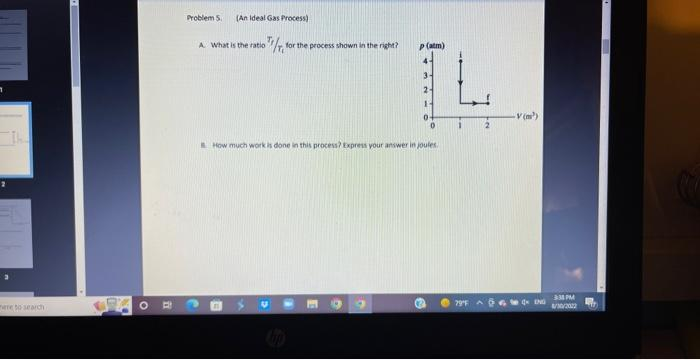# (Solved): need help answering Q5b A. What is the ratio $$f / \mathrm{T}_{\mathrm{f}}$$ ior the process shown ...A. What is the ratio $$f / \mathrm{T}_{\mathrm{f}}$$ ior the process shown in the right? How much work in done in this procens? Express your answer in joules.